# Texas Go Math Grade 4 Lesson 7.3 Answer Key Multiply Using the Distributive Property

Refer to our Texas Go Math Grade 4 Answer Key Pdf to score good marks in the exams. Test yourself by practicing the problems from Texas Go Math Grade 4 Lesson 7.3 Answer Key Multiply Using the Distributive Property.

## Texas Go Math Grade 4 Lesson 7.3 Answer Key Multiply Using the Distributive Property

Essential Question

How can you use the Distributive Property to multiply a 2-digit number by o I-digit number?
Explanation:
Here, we can use the Distributive property by multiplying a sum by a number is the same as multiplying each addend by the number and adding the products.

Investigate

Materials

• color pencils
• grid paperYou can use the Distributive Property to break apart numbers to make them easier to multiply.

The Distributive Property states that multiplying a sum by a number is the same as multiplying each addend by the number and then adding the products.

A. Outline a rectangle on the grid to model 6 × 13.
Here, we have outlined a rectangle on the grid to the model 6 × 13B. Think of 13 as 5 + 8. Break apart the model to show 6 × (5 + 8). Label and shade the smaller rectangles. Use two different colors.

Use the Distributive Property. Find the product each smaller rectangle represents. Then find the sum of the products. Record your answers._____________ × _____________ = _____________
_____________ × _____________ = _____________
_____________ + _____________ = _____________
6 × 13 = 78.

Explanation:
Here, by using Distributive Property we will break apart 6 × 13 as 6 × (5 + 8),
So 6 × 13 = 6 × (5 + 8)
= (6 × 5)+(6 × 8)
= 30 + 48
= 78.

C. Model 6 × 13 again. Think of 13 as a different sum. Break apart the model to show 6 × ( _____________ + _____________). Find the product each smaller rectangle represents. Then find the sum of the products. Record your answers.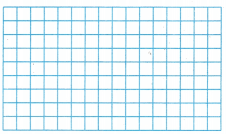_____________ × _____________ = _____________
_____________ × _____________ = _____________
_____________ + _____________ = _____________
6 × 13 = 78.

Explanation:
Here, by using Distributive Property we will model and break apart 6 × 13 as 6 × (5 + 8),
So 6 × 13 = 6 × (5 + 8)
= (6 × 5)+(6 × 8)
= 30 + 48
= 78.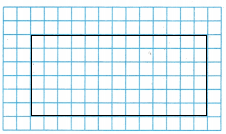Make Connections

Another way to model the problem is to use base-ten blocks to show tens and ones.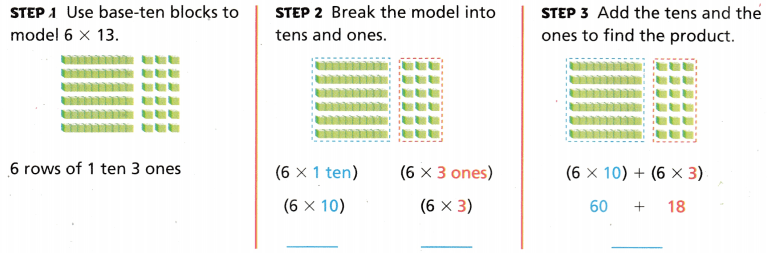So, 6 × 13 = 78.

In Step 2, the model is broken into two parts. Each part shows a partial product. The partial products are 60 and 18.
Here, the model is broken into two parts and each part shows a partial product so the partial products are 60 and 18.Math Talk

Mathematical Processes
How does breaking apart the model into tens and ones make finding the product easier?
By breaking apart the model into tens and ones we can add the tens and the ones to find the product.

Share and Show

Model the product on the grid. Record the product.

Question 1.
5 × 14 = ___________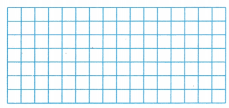5 × 14 = 70.

Explanation:
Here, we have used the distributive property for 5 × 14 and modeled the rectangle. So 5 × 14 is
5 × 14 = 5 ×(10+4)
= (5 × 10)+(5 × 4)
= 50 + 20
= 70.

Question 2.
4 × 16 = ___________Explanation:
By using the distributive property for 4 × 16 which is
4 × 16 = 4 × (10+6)
= (4×10) + (4×6)
= 40+24
= 64.

Use grid paper or base-ten blocks to model the product.
Then record the product.

Question 3.
7 × 12 = ___________
7 × 12 = 84.

Explanation:
By using the distributive property for 7 × 12 which is
7 × 12 = 7 × (10+2)
= (7 × 10) + (7 × 2)
= 70+14
= 84.

Question 4.
5 × 16 = ___________
5 × 16 = 80.

Explanation:
By using the distributive property for 5 × 16 which is
5 × 16 = 5 × (10+6)
= (5 × 10) + (5 × 6)
= 50+30
= 80.

Question 5.
9 × 13 = ___________
9 × 13 = 117.

Explanation:
By using the distributive property for 9 × 13 which is
9 × 13 = 9 ×(10+3)
= (9 × 10) + (9 × 3)
= 90+27
= 117.

Question 6.
H.O.T. Evaluate To find 7 × 23, is it easier to break apart the factor, 23, as 20 + 3 or 15 + 8? Explain.

Problem Solving

H.O.T. Pose a ProblemQuestion 7.
Evaluate Kyle went to a fruit market. The market sells a wide variety of fruits and vegetables. The picture at the right shows a display of oranges.Write a problem that can be solved using the picture.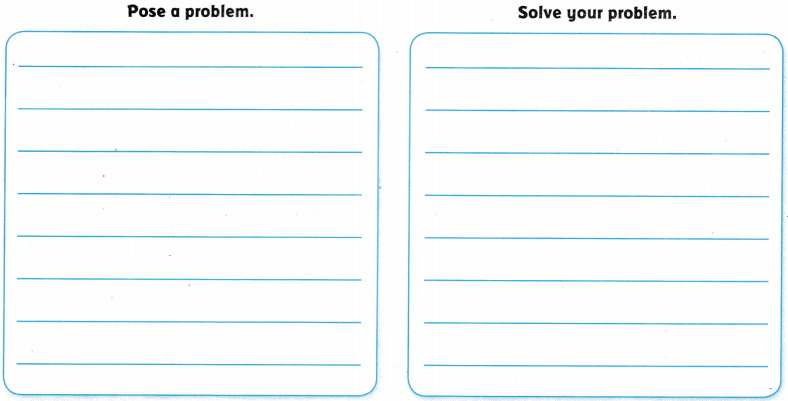Question 8.
H.O.T. Multi-Step Use Math Language Describe how you could change the problem by changing the number of rows of oranges and the number of empty spaces in the picture. Then solve the problem.

Question 9.
Ryan plays with his robo-pup 17 minutes each day. How many minutes will Ryan play with his robo-pup in 5 days?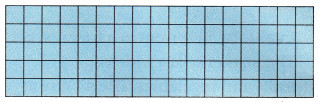(A) 34 minutes
(B) 22 minutes
(C) 57 minutes
(D) 85 minutes
D.

Explanation:
Given that Ryan plays with his Robo-pup for 17 minutes each day. So the number of minutes will Ryan play with his Robo-pup in 5 days is 17×5 which is 85 minutes.

Question 10.
There are 13 muffins in each box. Which shows the total number of muffins in 4 boxes?(A) (4 × 10) + (4 × 3) = 52
(B) (4 × 10) + 3 = 43
(C) 4 + (10 × 3) = 34
(D) (4 + 10) +(4 + 3) = 21
A.

Explanation:
Given that there are 13 muffins in each box, so the total number of muffins in 4 boxes is (4 × 10) + (4 × 3) = 52.

Question 11.
Multi-Step Ava and Mia are setting up chairs for the play. Ava sets up 6 rows of chairs with 14 chairs in each row. Mia sets up 5 rows of chairs with 16 chairs in each row. How many more chairs does Ava set up than Mia?
(A) 4
(B) 5
(C) 6
(D) 14
A.

Explanation:
Given that Ava and Mia are setting up chairs for the play and Ava sets up 6 rows of chairs with 14 chairs in each row which is 6×14 = 84 chairs and Mia sets up 5 rows of chairs with 16 chairs in each row which is 5×16 = 80 chairs. So the number of chairs does Ava set up Mia is 84-80 which is 4.

TEXAS Test Prep

Question 12.
Brenda has already handed out 17 programs for the play. Before the play is over, she needs to have handed out a total that is 4 times that many programs. How many programs does Brenda have left to hand out before the end of the play?
(A) 21
(B) 63
(C) 80
(D) 51
A.

Explanation:
Given that Brenda has already handed out 17 programs for the play and before the play is over, she needs to have handed out a total that is 4 times that many programs. So the number of programs does Brenda has left to hand out before the end of the play is 17+4 which is 21.

### Texas Go Math Grade 4 Lesson 7.3 Homework and Practice Answer Key

Model the problem on the grid. Record the product.

Question 1.
7 × 13 = _____________7 × 13 = 91.

Explanation:
The product of 7 × 13 is 91.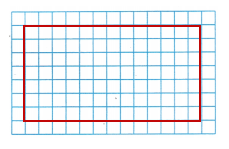Question 2.
5 × 15 = ______________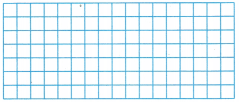5 × 15 = 75.

Explanation:
The product of 5 × 15 is 75.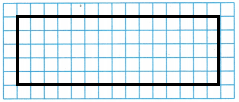Use grid paper or base-ten blocks to model the product.
Then record the product.

Question 3.
3 × 14 = ______________
3 × 14 = 42.

Explanation:
The product of 3 × 14 is 42.

Question 4.
8 × 16 = ______________
8 × 16 = 128.

Explanation:
The product of 8 × 16 is 128.

Question 5.
9 × 12 = ______________
9 × 12 = 108.

Explanation:
The product of 9 × 12 is 108.

Question 6.
5 × 14 = ______________
5 × 14 = 70.

Explanation:
The product of 5 × 14 = 70.

Question 7.
7 × 11 = ______________
7 × 11 = 77.

Explanation:
The product of 7 × 11 = 77.

Question 8.
2 × 18 = ______________
2 × 18 = 36.

Explanation:
The product of 2 × 18 = 36.

Problem Solving

Jeannie has a flat of daisies. She has planted some of them in her garden.

Write a problem that can be solved using the picture.Question 9.
Pose a problem.

Question 10.
Solve a problem.

Lesson Check

Question 11.
The shaded part of the model shows how Jose tiled his floor.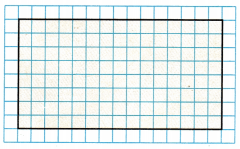How many tiles did Jose use?
(A) 120
(B) 160
(C) 80
(D) 140
A.

Explanation:
The number of tiles did Jose use is 8×15 which is 120.

Question 12.
The model below represents the expression 6 × 16.How many tens will there be in the final product?
(A) 4
(B) 3
(C) 9
(D) 7
C.

Explanation:
As the model represents 6 × 16 which is 96, so the number of tens in the final product is 9.

Question 13.
Will collects military medals. He has 5 cases of medals. Each case holds 18 medals. How many medals does Will have?
(A) 75
(B) 95
(C) 80
(D) 90
D.

Explanation:
Given that Will collects military medals and he has 5 cases of medals. Each case holds 18 medals, so the number of medals does Will has is 5×18 which is 90.

Question 14.
Yolando has 12 inches of ribbon. She needs 6 times as much ribbon than what she has. How many inches of ribbon does Yolanda need?
(A) 74 inches
(B) 62 inches
(C) 60 inches
(D) 72 inches
D.

Explanation:
Given that Yolando has 12 inches of ribbon and she needs 6 times as much ribbon as what she has. So Yolanda need is 12×6 which is 72 inches.

Question 15.
There are 24 bottles of water in a case. Coach Rinaldo buys 4 cases of water for the school field day. How many bottles did Coach Rinaldo buy?
(A) 86
(B) 96
(C) 88
(D) 90
B.

Explanation:
Given that there are 24 bottles of water in a case and coach Rinaldo buys 4 cases of water for the school field day. So the number of bottles did Coach Rinaldo bought is 24×4 which is 96.

Question 16.
Multi-Step Peyton bought 4 cartons of eggs with 12 eggs in each carton. Chloe bought 3 cartons of eggs with 18 eggs in each carton. Which statement is true?
(A) Chloe bought more eggs than Peyton.
(B) Together Chloe and Peyton bought 96 eggs.
(C) Peyton bought 6 more eggs than Chloe.
(D) Both girls bought the same number of eggs.